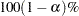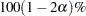Displayed Output

For an AB/BA crossover design, the "CrossoverVarInfo" table shows the variables specified for the response and treatment values in each period of the design.

The summary statistics in the "Statistics" table and confidence limits in the "ConfLimits" table are displayed for certain variables and/or transformations or subgroups of the variables in the analysis, depending on the design. For a one-sample design, summary statistics are displayed for all variables in the analysis. For a paired design, statistics are displayed for the difference if you specify the TEST=DIFF option in the PROC TTEST statement, or for the ratio if you specify TEST=RATIO. For a two-independent-sample design, the statistics for each of the two groups and for the difference (if TEST=DIFF) or ratio (if TEST=RATIO) are displayed. For an AB/BA crossover design, statistics are displayed for each of the four cells in the design (all four combinations of the two periods and two treatments). If the IGNOREPERIOD option is absent, then if TEST=DIFF is specified, statistics are displayed for the treatment difference within each sequence and overall, and also for the period difference. If TEST=RATIO, statistics are displayed for the treatment ratio within each sequence and overall, and also for the period ratio. If the IGNOREPERIOD option is specified in the VAR statement, then statistics are displayed for the overall treatment difference if TEST=DIFF or for the overall treatment ratio if TEST=RATIO.

The "Statistics" table displays the following summary statistics:

• the name of the variable(s), displayed if the NOBYVAR option is used in the PROC TTEST statement

• the name of the classification variable (if two-independent-sample design) or treatment and period (if AB/BA crossover design)

• N, the number of nonmissing values

• the (arithmetic) Mean, displayed if the DIST=NORMAL option is specified in the PROC TTEST statement

• the Geometric Mean, displayed if the DIST=LOGNORMAL option is specified in the PROC TTEST statement

• Std Dev, the standard deviation, displayed if the DIST=NORMAL option is specified in the PROC TTEST statement

• the Coefficient of Variation, displayed if the DIST=LOGNORMAL option is specified in the PROC TTEST statement

• Std Err, the standard error of the mean, displayed if the DIST=NORMAL option is specified in the PROC TTEST statement

• the Minimum value

• the Maximum value

The "ConfLimits" table displays the following:

• the name of the variable(s), displayed if the NOBYVAR option is used in the PROC TTEST statement

• the name of the classification variable (if two-independent-sample design) or treatment and period (if AB/BA crossover design)

• the (arithmetic) Mean, displayed if the DIST=NORMAL option is specified in the PROC TTEST statement

• the Geometric Mean, displayed if the DIST=LOGNORMAL option is specified in the PROC TTEST statement

•CL Mean, the lower and upper confidence limits for the mean. Separate pooled and Satterthwaite confidence limits are shown for the difference or ratio transformations in two-independent-sample designs and AB/BA crossover designs without the IGNOREPERIOD option.

• Std Dev, the standard deviation, displayed if the DIST=NORMAL option is specified in the PROC TTEST statement

• the Coefficient of Variation, displayed if the DIST=LOGNORMAL option is specified in the PROC TTEST statement

•CL Std Dev, the equal-tailed confidence limits for the standard deviation, displayed if the DIST=NORMAL and CI=EQUAL options are specified in the PROC TTEST statement

•UMPU CL Std Dev, the UMPU confidence limits for the standard deviation, displayed if the DIST=NORMAL and CI=UMPU options are specified in the PROC TTEST statement

•CL CV, the equal-tailed confidence limits for the coefficient of variation, displayed if the DIST=LOGNORMAL and CI=EQUAL options are specified in the PROC TTEST statement

•UMPU CL CV, the UMPU confidence limits for the coefficient of variation, displayed if the DIST=LOGNORMAL and CI=UMPU options are specified in the PROC TTEST statement

The confidence limits in the "EquivLimits" table and test results in the "TTests" and "EquivTests" tables are displayed only for the test criteria—that is, the variables or transformations being tested. For a one-sample design, results are displayed for all variables in the analysis. For a paired design, results are displayed for the difference if you specify the TEST=DIFF option in the PROC TTEST statement, or for the ratio if you specify TEST=RATIO. For a two-independent-sample design, the results for the difference (if TEST=DIFF) or ratio (if TEST=RATIO) are displayed. For an AB/BA crossover design, results are displayed for the treatment difference (if TEST=DIFF) or ratio (if TEST=RATIO). If the IGNOREPERIOD option is absent, then results are also displayed for the period difference (if TEST=DIFF) or ratio (if TEST=RATIO).

The "EquivLimits" table, produced only if the TOST option is specified in the PROC TTEST statement, displays the following:

• the name of the variable(s), displayed if the NOBYVAR option is used in the PROC TTEST statement

• the (arithmetic) Mean, displayed if the DIST=NORMAL option is specified in the PROC TTEST statement

• the Geometric Mean, displayed if the DIST=LOGNORMAL option is specified in the PROC TTEST statement

• Lower Bound, the lower equivalence bound for the mean specified in the TOST option in the PROC TTEST statement

•CL Mean, the lower and upper confidence limits for the mean relevant to the equivalence test. Separate pooled and Satterthwaite confidence limits are shown for two-independent-sample designs and AB/BA crossover designs without the IGNOREPERIOD option.

• Upper Bound, the upper equivalence bound for the mean specified in the TOST option in the PROC TTEST statement

• Assessment, the result of the equivalence test at the significance level specified by the ALPHA= option in the PROC TTEST statement, either "Equivalent" or "Not equivalent"

The "TTests" table is produced only if the TOST option is not specified in the PROC TTEST statement. Separate results for pooled and Satterthwaite tests (and also the Cochran and Cox test, if the COCHRAN option is specified in the PROC TTEST statement) are displayed for two-independent-sample designs and AB/BA crossover designs without the IGNOREPERIOD option. The table includes the following results:

• the name of the variable(s), displayed if the NOBYVAR option is used in the PROC TTEST statement

• t Value, thestatistic for comparing the mean to the null value as specified by the H0= option in the PROC TTEST statement

• DF, the degrees of freedom

• the-value, the probability of obtaining astatistic at least as extreme as the observedvalue under the null hypothesis

The "EquivTests" table is produced only if the TOST option is specified in the PROC TTEST statement. Separate results for pooled and Satterthwaite tests are displayed for two-independent-sample designs and AB/BA crossover designs without the IGNOREPERIOD option. Each test consists of two separate one-sided tests. The overall-value is the larger-value from these two tests. The table includes the following results:

• the name of the variable(s), displayed if the NOBYVAR option is used in the PROC TTEST statement

• Null, the lower equivalence bound for the Upper test or the upper equivalence bound for the Lower test, as specified by the TOST option in the PROC TTEST statement

• t Value, thestatistic for comparing the mean to the Null value

• DF, the degrees of freedom

• the-value, the probability of obtaining astatistic at least as extreme as the observedvalue under the null hypothesis

The "Equality" table gives the results of the test of equality of variances. It is displayed for two-independent-sample designs and AB/BA crossover designs without the IGNOREPERIOD option. The table includes the following results:

• the name of the variable(s), displayed if the NOBYVAR option is used in the PROC TTEST statement

• Num DF and Den DF, the numerator and denominator degrees of freedom

• F Value, the(folded) statistic

• Pr > F, the probability of a greatervalue. This is the two-tailed-value.